# Simulating and cleaning gene expression data using RUVcorr in the context of inferring gene co-expression

The R package RUVcorr allows the simulation and cleaning of gene expression data using global removal of unwanted variation (RUV) when the aim is the inference of gene co-expression. Besides the RUV procedure, the package offers extensive plotting options related to its application and can simulate realistic gene expression data with a known gene correlation structure. Although the procedures in the RUVcorr package have so far only been applied to microarray gene expression data it should be feasible to apply it to RNA-seq data as well, as long as suitable read-count summaries have been generated and the coverage is sufficient, however this remains untested.

Loading RUVcorr is achieved with the command:

library(RUVcorr)

# Simulating gene expression data with a known gene correlation structure

The simulation of gene expression data relies on the linear model framework introduced by Gagnon-Bartsch and Speed. Briefly, they assume that any gene expression measurement can be expressed as a linear combination of biological signal $$X\beta$$, systematic noise $$W\alpha$$, and random noise $$\epsilon$$ (typically assumed to be iid normally distributed).

$\begin{equation} Y=X\beta+W\alpha+\epsilon \end{equation}$
• $$Y$$ is a $$m \times n$$ matrix of observed gene expression data
• $$X$$ is a $$m \times p$$ matrix containing the unobserved factors of interest
• $$\beta$$ is a $$p \times n$$ matrix of regression coefficients associated with $$X$$
• $$W$$ is a $$m \times k$$ matrix of unobserved covariates introducing systematic noise
• $$\alpha$$ is a $$k \times n$$ matrix of regression coefficients associated with $$W$$
• $$\epsilon$$ is a $$m \times n$$ matrix of random noise

In the context of this model and for the purposes of simulating gene expression data with a known gene correlation structure, the true underlying gene structure is assumed to be $$\Sigma=Cor(X\beta)$$. The size of the absolute value of the correlations can be somewhat controlled using the dimensionality of $$X$$ and $$\beta$$, $$p$$. When $$p$$ is increased the size of the absolute value of the correlations in $$\Sigma$$ is reduced. Note that some genes (negative controls) are unaffected by this, as their correlation with each other as well as other genes is defined to be 0. Negative control genes are genes that are believed to be unrelated to the factor of interest.

## Independence of biological signal and systematic noise

The simplest simulation of gene expression data assumes that the biological signal and the systematic noise are uncorrelated with each other. So $$X$$ is simulated in a fashion that it renders it independent from $$W$$. After simulating the data, the print command allows you to get a useful overview of the simulated data as well as some meta data.

set.seed(400)
Yind <- simulateGEdata(n=3000, m=1000, k=10, size.alpha=2,
corr.strength=5, g=NULL, Sigma.eps=0.1,
nc=2000, ne=1000, intercept=TRUE, check=TRUE)
print(Yind)
## Simulated Data:
## Number of samples:  1000
##
## Number of genes:  3000
##
## Info:      [,1]               [,2]
## [1,] "k"                "10"
## [2,] "Mean correlation" "0.37367"
## [3,] "Size alpha"       "2"
## [4,] "Intercept"        "1"
##
##
##  Truth
##          [,1]     [,2]     [,3]     [,4]     [,5]
## [1,] 3.447821 3.798722 12.87851 13.89850 4.212844
## [2,] 3.855001 3.674299 13.04912 14.05321 3.763207
## [3,] 4.124890 4.027222 12.75715 13.81689 3.461644
## [4,] 4.096000 3.858704 13.20210 14.52872 3.619737
## [5,] 4.274382 4.007947 13.00307 14.22137 3.381000
##
##
##  Y
##           [,1]     [,2]     [,3]      [,4]      [,5]
## [1,] 0.9178217 3.144494 13.12859 15.618884 0.5393862
## [2,] 3.1760868 2.369838 13.37485 12.801848 5.4446971
## [3,] 3.5460899 7.921163 13.06658 16.142114 7.1591649
## [4,] 5.9339592 2.374305 14.50340  6.472955 4.1597942
## [5,] 2.6708657 5.704914  9.08178 10.086742 5.5447521
##
##
##  Noise
##            [,1]       [,2]       [,3]      [,4]       [,5]
## [1,] -2.3963091 -0.5719278  0.1424810  1.644274 -3.6232646
## [2,] -0.6583354 -1.4789223  0.3642906 -1.316678  1.6108319
## [3,] -0.5895675  4.0725809  0.3620272  2.399870  3.8290098
## [4,]  1.8112245 -1.5876917  1.2016046 -8.077589  0.5674677
## [5,] -1.5802649  1.6729883 -3.8756275 -4.200470  2.2899458
##
##
##  Sigma
##            [,1]       [,2]       [,3]       [,4]       [,5]
## [1,]  1.0000000  0.2300757  0.7077388  0.5133700 -0.3523293
## [2,]  0.2300757  1.0000000 -0.2131211  0.1073550  0.1087782
## [3,]  0.7077388 -0.2131211  1.0000000  0.4973006  0.1772923
## [4,]  0.5133700  0.1073550  0.4973006  1.0000000 -0.2652279
## [5,] -0.3523293  0.1087782  0.1772923 -0.2652279  1.0000000

Note that the parameter corr.strength refers to $$p$$. The parameters nc and ne refer to the number of negative control genes and truly expressed genes (i.e. with a mean true gene expression greater than 0.) The parameter intercept controls whether $$W$$ contains an offset or not.

#Dependence of biological signal and systematic noise

It is more realistic to assume that there is some dependence between $$X$$ and $$W$$. Using the parameter g ($$0<g \leq \min(k,p)$$) it is possible to introduce different levels of correlation between signal and systematic noise. Choosing a larger value for g will introduce more dependency between $$X$$ and $$W$$. Here g refers to the dimension of the shared subspace of $$X$$ and $$W$$.

set.seed(400)
Ydep <- simulateGEdata(n=3000, m=1000, k=10, size.alpha=2,
corr.strength=5, g=2, Sigma.eps=0.1,
nc=2000, ne=1000, intercept=TRUE, check=TRUE)
##  "Need to make positive semi-definite!"
print(Ydep)
## Simulated Data:
## Number of samples:  1000
##
## Number of genes:  3000
##
## Info:      [,1]               [,2]
## [1,] "k"                "10"
## [2,] "Mean correlation" "0.37405"
## [3,] "bWX"              "0.17538"
## [4,] "Size alpha"       "2"
## [5,] "Intercept"        "1"
##
##
##  Truth
##           [,1]     [,2]     [,3]     [,4]     [,5]
## [1,] 10.143886 15.53114 2.546970 3.226404 14.89994
## [2,]  9.068776 14.99081 1.582244 1.922911 14.57282
## [3,] 10.503293 15.05288 1.728851 3.292824 12.37187
## [4,] 11.535333 15.92762 2.611494 3.567288 13.36926
## [5,] 11.234852 14.50556 2.998051 2.525897 13.31959
##
##
##  Y
##           [,1]     [,2]      [,3]        [,4]      [,5]
## [1,]  9.398858 12.59123 0.7883212 -0.63822024 15.857143
## [2,] 10.044989 14.27661 1.1739130 -1.00930559 12.391147
## [3,]  8.819857 12.60346 2.4811734  0.05810922 13.831401
## [4,] 15.546464 14.65987 7.2123839 -0.14543726 13.222961
## [5,] 14.562392 16.68100 3.7431212  1.78321722  9.399726
##
##
##  Noise
##            [,1]       [,2]       [,3]       [,4]       [,5]
## [1,] -0.6627286 -3.0475014 -1.8347581 -3.8144307  1.1043862
## [2,]  0.8017511 -0.6756356 -0.4736520 -3.0028747 -2.0142852
## [3,] -1.5047964 -2.3968253  0.8269651 -3.1032253  1.6194624
## [4,]  3.9078375 -1.3674478  4.5790674 -3.6853142 -0.3203399
## [5,]  3.3035614  2.2211075  0.6792264 -0.6164858 -3.8738138
##
##
##  Sigma
##            [,1]       [,2]       [,3]       [,4]       [,5]
## [1,]  1.0000000  0.1855021  0.6649428  0.4539661 -0.4180683
## [2,]  0.1855021  1.0000000 -0.2848953  0.1226356  0.1077881
## [3,]  0.6649428 -0.2848953  1.0000000  0.4802257  0.1512601
## [4,]  0.4539661  0.1226356  0.4802257  1.0000000 -0.1990134
## [5,] -0.4180683  0.1077881  0.1512601 -0.1990134  1.0000000

Note that bWX refers to the average correlation between the columns of $$X$$ and $$W$$.

# Application of global removal of unwanted variation

RUV is a data-driven method that removes systematic noise from gene expression datasets. The particular version of RUV is dependent on the goal of the analysis. We have developed a method, RUVNaiveRidge, for the removal of unwanted variation that focuses on retrieving the true underlying gene-gene correlations, but at the cost of the specification of the absolute values of gene expression.The application of RUVNaiveRidge requires the analyst to make several descisions, which should be informed by the ultimate research goal. Here we will demonstrate some of the principles using a dataset on gene expression in 57 samples from the bladder as described in Dyrskjot et al. The dataset can be found in the Bioconductor package bladderbatch. Note that this dataset is small and co-expression analysis should ideally be performed on studies with at least 100 samples.

## Investigating the dataset design and getting data into the correct format

For the application of RUVNaiveRidge it is important to be familar with the experiment design of the dataset. If the accompanying metadata of the samples is available the experiment design can be visualized using the function plotDesign.

library(bladderbatch)
plotDesign(expr.meta, c("cancer", "outcome", "batch"),
c("Diagnosis", "Outcome", "Batch"), orderby="batch")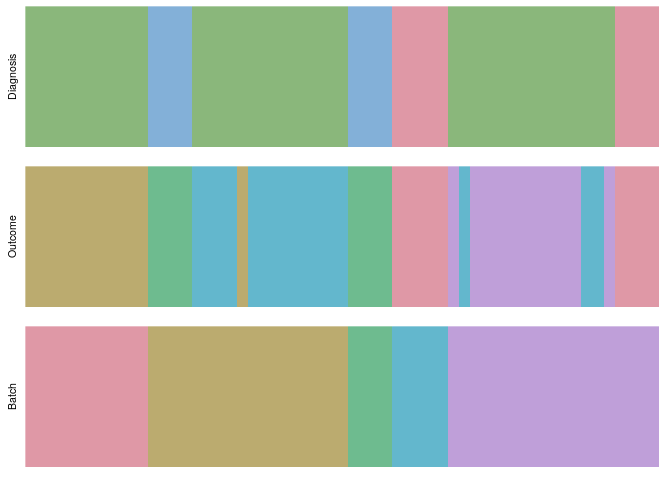Every line in each of the bars represents a sample, which is colored according to the factor displayed on the left-hand side. The samples in each bar are in the same order

The figure illustrates that batches, diagnosis and eventual outcome were substantially confounded; ie. not all factors could be fully randomized. Thus, it is likely that the data contains some systematic noise.

The gene expression data needs to be a matrix with its columns containing the genes and its rows containing the samples.

expr <- exprs(bladderEset)
expr[1:5,1:5]
##           GSM71019.CEL GSM71020.CEL GSM71021.CEL GSM71022.CEL GSM71023.CEL
## 1007_s_at    10.115170     8.628044     8.779235     9.248569    10.256841
## 1053_at       5.345168     5.063598     5.113116     5.179410     5.181383
## 117_at        6.348024     6.663625     6.465892     6.116422     5.980457
## 121_at        8.901739     9.439977     9.540738     9.254368     8.798086
## 1255_g_at     3.967672     4.466027     4.144885     4.189338     4.078509
dim(expr)
##  22283    57
expr <- t(expr)
expr <- expr[,1:20000]

library(hgu133a2.db)
x <- hgu133a2SYMBOL
xx <- as.list(x[colnames(expr)])

## Selecting negative control genes

Ideally, negative control genes should be selected with the help of a priori information. Unfortunately, when the aim is estimating gene coexpression and the factor of interest is unknown, a suitable set of negative control genes is seldomly known. Because of this it is advisable to choose negative control genes empirically. Using the RUVcorr package this can be accomplished using the function empNegativeControls. Note that it is necessary to exclude the genes that pertain to your research question from being selected as negative controls. For demonstration purposes let us assume we are interested in the following 10 random genes:

na_genes <- c("SCN1A", "SCN3A", "SCN4A", "SCN5A", "SCN7A", "SCN8A", "SCN11A",
"SCN1B", "SCN2B", "SCN3B", "SCN4B")

Since the genes in the dataset is uing Affymetrix identifiers, we have to find the corresponding Affymetrix probe names for our genes of interest.

na_affy <- names(which(unlist(lapply(xx, function(x) is.element(x, na_genes)))))
na_index <- which(is.element(colnames(expr),na_affy))
nc_index <- empNegativeControls(expr, exclude=na_index, nc=3000)

Usefully, the selection can also be visualized:

genePlot(expr, index=nc_index,
legend="Negative Control Genes", title="IQR-Mean Plot")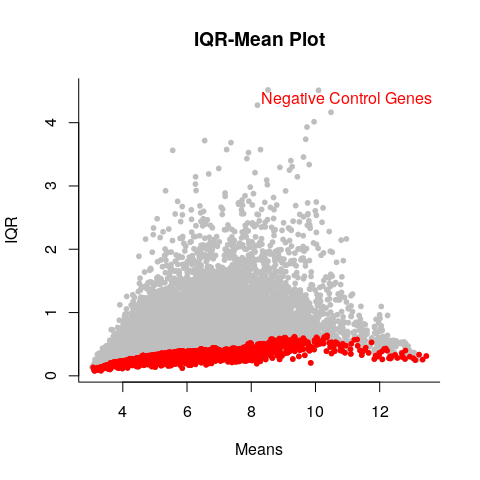This figure shows the inter-quantile range vs. mean plot of the expression of all genes. The genes highlighted in red are the empirically chosen negative control genes.

## Effective application of RUVNaiveRidge

Besides negative control genes the application of RUVNaiveRidge also requires the input of two user-selected parameters, the ridge parameter $$\nu$$ and the dimensionality of $$\hat{W}$$, $$\hat{k}$$. Since these parameters determine the strength of the cleaning, the user is adviced to carefully assess her choices. It is recommended to run RUVRidgeNaive with several different choices of the parameters and then assess the results. In order to do this efficiently it is advisable to RUVNaiveRidge in parallel. This can be achieved with a package such as snowfall.

library(snowfall)
k <- c(1,2,3,4)
nu <- c(0,500,1000,5000)
k.nu.matrix <- cbind(rep(k, each=4), rep(nu, 4))
k.nu.matrix <- as.list(as.data.frame(t(k.nu.matrix)))

sfInit(parallel=TRUE, cpus=4)
## R Version:  R version 4.0.0 (2020-04-24)
sfLibrary(RUVcorr)
## Library RUVcorr loaded.
sfExport("expr", "k.nu.matrix", "nc_index")
expr_AllRUV <- sfLapply(k.nu.matrix, function(x)
RUVNaiveRidge(expr, center=TRUE, nc_index, x, x))
sfStop()

## Plotting options to help make parameter choices

Choosing the parameter values is not always easy and there might be more than one possible choice. It is therefore vital to thoroughly investigate different combinations of parameter choices using genes that are a prior} known to be uncorrelated with each other and a priori known to be correlated, also referred to as positive controls. Here, we will use the sodium channel genes as positive controls, because we expect some of these genes to be correlated with each other.

cor_AllRUV_na <- lapply(expr_AllRUV, function(x) cor(x[,na_index]))
cor_Raw_na <- cor(expr[,na_index])

lapply(1:4, function(i) histogramPlot(cor_AllRUV_na[seq(0,15,4)+i], cor_Raw_na,
title=paste("nu=", nu[i]), legend=c(paste("k=", k), "Raw")))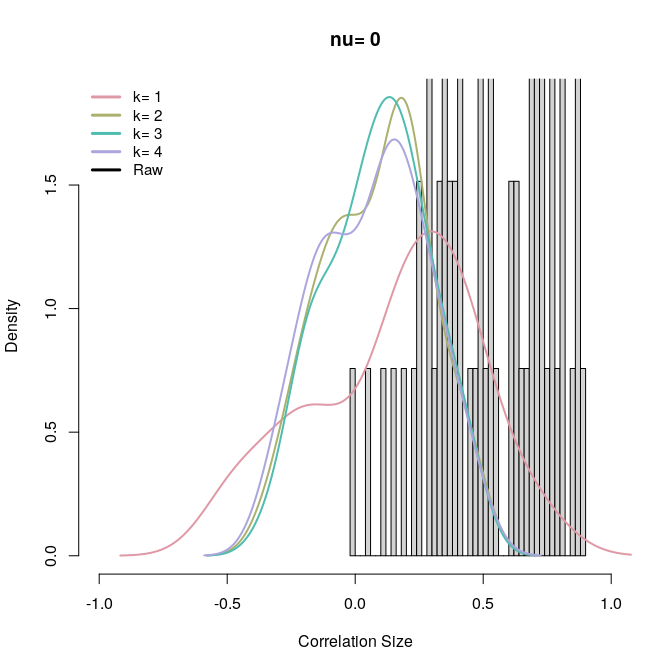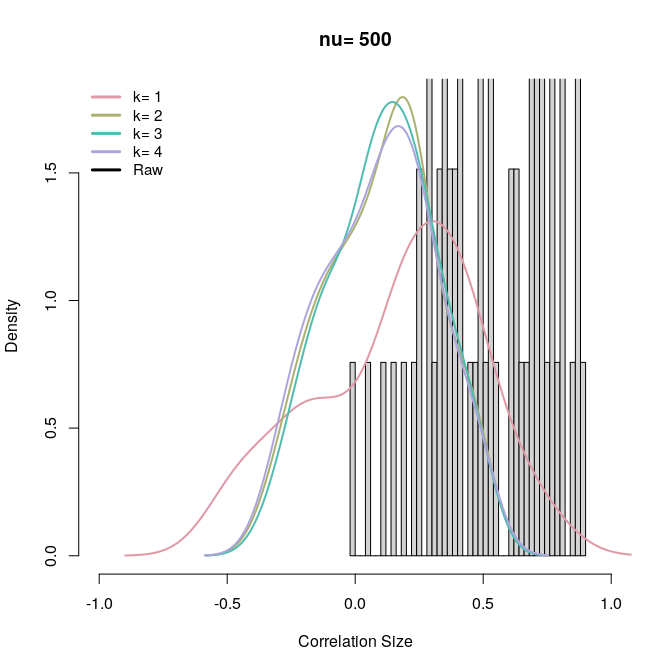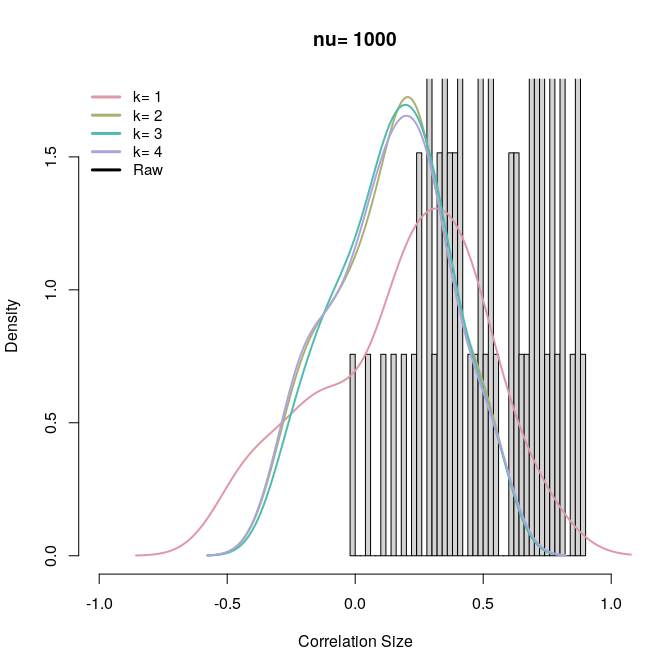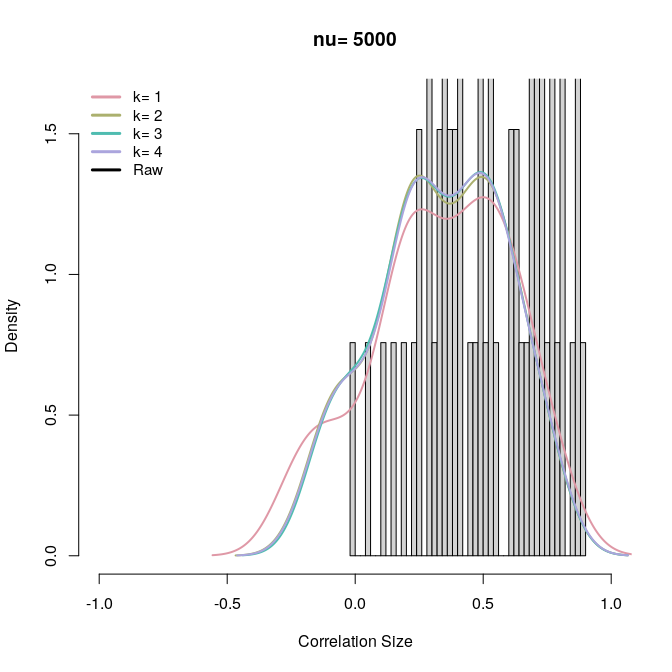## []
## []$rect ## []$rect$w ##  0.3576563 ## ## []$rect$h ##  0.4428297 ## ## []$rect$left ##  -1.08 ## ## []$rect$top ##  1.930151 ## ## ## []$text
## []$text$x
##  -0.86625 -0.86625 -0.86625 -0.86625 -0.86625
##
## []$text$y
##  1.856346 1.782541 1.708736 1.634931 1.561126
##
##
##
## []
## []$rect ## []$rect$w ##  0.3576563 ## ## []$rect$h ##  0.4288303 ## ## []$rect$left ##  -1.08 ## ## []$rect$top ##  1.869132 ## ## ## []$text
## []$text$x
##  -0.86625 -0.86625 -0.86625 -0.86625 -0.86625
##
## []$text$y
##  1.797660 1.726188 1.654716 1.583245 1.511773
##
##
##
## []
## []$rect ## []$rect$w ##  0.3576563 ## ## []$rect$h ##  0.4115874 ## ## []$rect$left ##  -1.08 ## ## []$rect$top ##  1.793976 ## ## ## []$text
## []$text$x
##  -0.86625 -0.86625 -0.86625 -0.86625 -0.86625
##
## []$text$y
##  1.725378 1.656780 1.588182 1.519584 1.450986
##
##
##
## []
## []$rect ## []$rect$w ##  0.3576563 ## ## []$rect$h ##  0.3890132 ## ## []$rect$left ##  -1.08 ## ## []$rect$top ##  1.695582 ## ## ## []$text
## []$text$x
##  -0.86625 -0.86625 -0.86625 -0.86625 -0.86625
##
## []$text$y
##  1.630746 1.565911 1.501075 1.436240 1.371404

For the set of uncorrelated genes, the negative control genes cannot be used. This is because negative controls used during RUV will have zero correlation by definition. A good choice for a set of uncorrelated genes is a set of random genes. Picking these can be accomplished using the function background.

bg_index <- background(expr, nBG=100, exclude=na_index, nc_index=nc_index)

cor_AllRUV_bg <- lapply(expr_AllRUV, function(x) cor(x[,bg_index]))
cor_Raw_bg <- cor(expr[,bg_index])

lapply(1:4, function(i) histogramPlot(cor_AllRUV_bg[seq(0,15,4)+i], cor_Raw_bg,
title=paste("nu=", nu[i]), legend=c(paste("k=", k), "Raw")))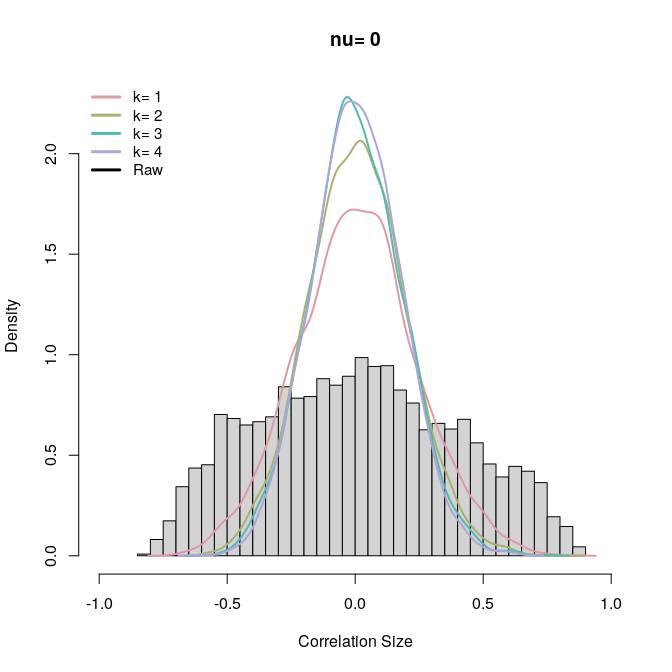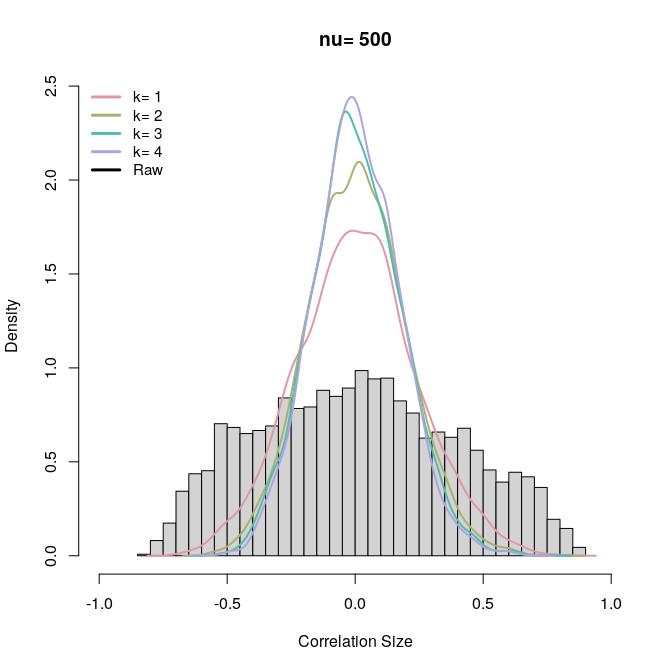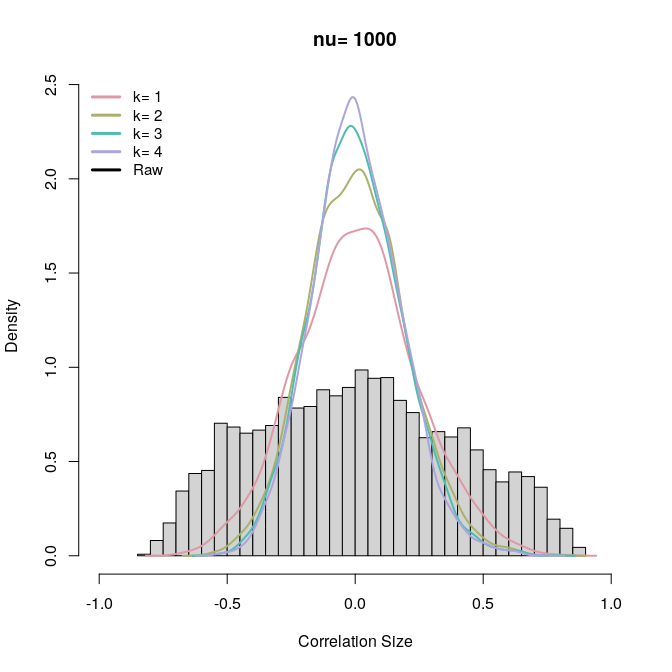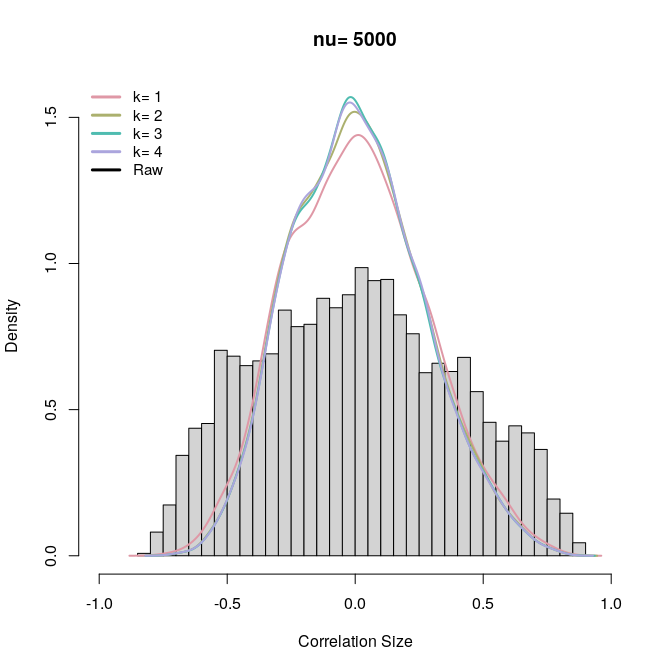## []
## []$rect ## []$rect$w ##  0.3576563 ## ## []$rect$h ##  0.5444101 ## ## []$rect$left ##  -1.08 ## ## []$rect$top ##  2.372906 ## ## ## []$text
## []$text$x
##  -0.86625 -0.86625 -0.86625 -0.86625 -0.86625
##
## []$text$y
##  2.282171 2.191436 2.100701 2.009966 1.919231
##
##
##
## []
## []$rect ## []$rect$w ##  0.3576563 ## ## []$rect$h ##  0.5825958 ## ## []$rect$left ##  -1.08 ## ## []$rect$top ##  2.539345 ## ## ## []$text
## []$text$x
##  -0.86625 -0.86625 -0.86625 -0.86625 -0.86625
##
## []$text$y
##  2.442246 2.345147 2.248047 2.150948 2.053849
##
##
##
## []
## []$rect ## []$rect$w ##  0.3576563 ## ## []$rect$h ##  0.5806968 ## ## []$rect$left ##  -1.08 ## ## []$rect$top ##  2.531068 ## ## ## []$text
## []$text$x
##  -0.86625 -0.86625 -0.86625 -0.86625 -0.86625
##
## []$text$y
##  2.434286 2.337503 2.240720 2.143937 2.047154
##
##
##
## []
## []$rect ## []$rect$w ##  0.3576563 ## ## []$rect$h ##  0.3744759 ## ## []$rect$left ##  -1.08 ## ## []$rect$top ##  1.632219 ## ## ## []$text
## []$text$x
##  -0.86625 -0.86625 -0.86625 -0.86625 -0.86625
##
## []$text$y
##  1.569806 1.507393 1.444981 1.382568 1.320155

The figures show the impact of different parameter choices on the correlations of a set of sodium channel genes and random genes. Correlation densities for different parameter choices. The histogram in the background of each panel shows the denisty of the correlations of the random genes calculated using the raw data.

From the figures it seems a choice of $$\hat{k}=2$$ corrects the wide range of the distribution of the correlations between random genes, but leaves some interesting non-zero correlations for the sodium-channel genes. Other plots that are informative for the choise of $$k$$ include the eigenvaluePlot. The choice for the correct $$\nu$$ however remains difficult because of the little change in the overall results. Further assessments are required.

Besides looking at histogram plots studying relative log expression (RLE) plots is useful. Specifically, parameter choices that overcorrect the data can be spotted. Such parameter choices will have gene expression variances that are too low. The RLE plots offered differ from the originally proposed RLE plot by combining all samples and are suited to large ($$>100$$ arrays) gene expression data sets where visualisation of individual arrays becomes impractical. The option displayed here shows the boxplots for the 1st and 3rd quantile of the difference between the gene expression and the study median for all samples.

lapply(1:4, function(i) RLEPlot(expr, expr_AllRUV[[4+i]],
name=c("Raw", "RUV"), title=paste("nu=", nu[i]),
method="IQR.boxplots"))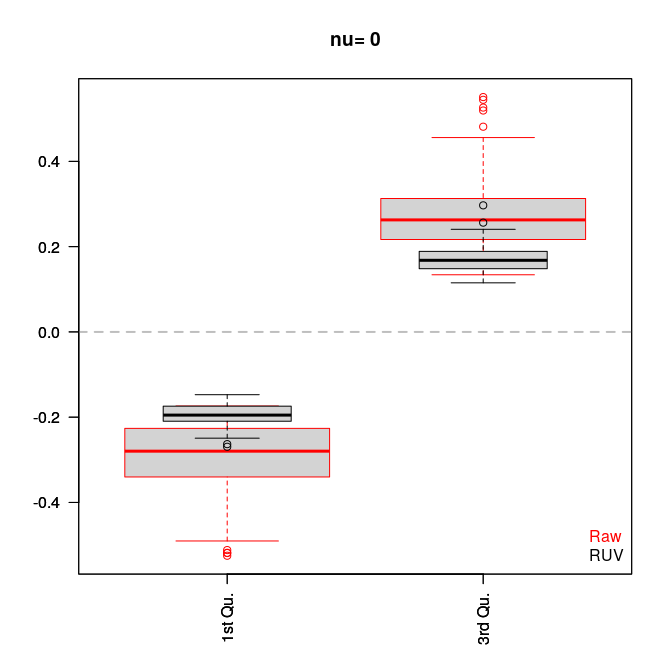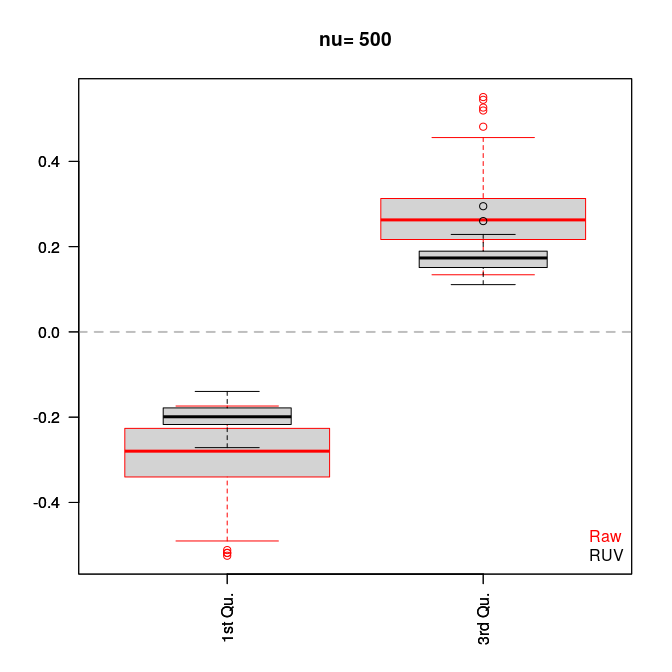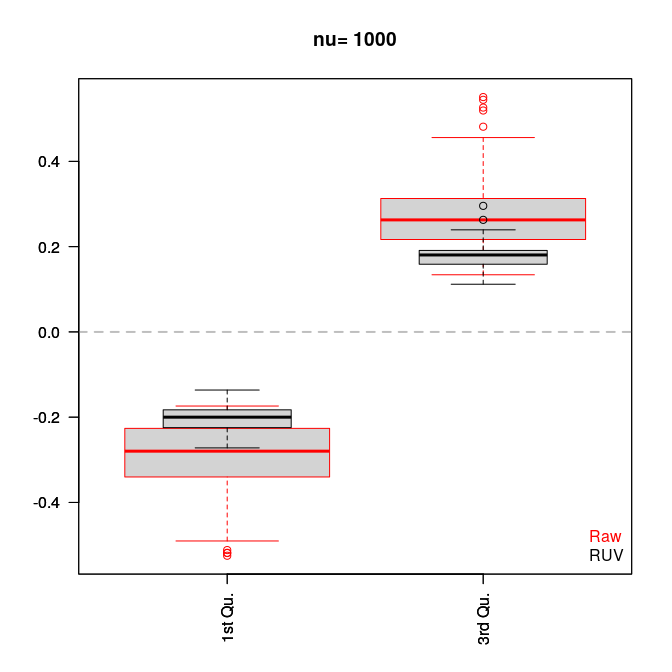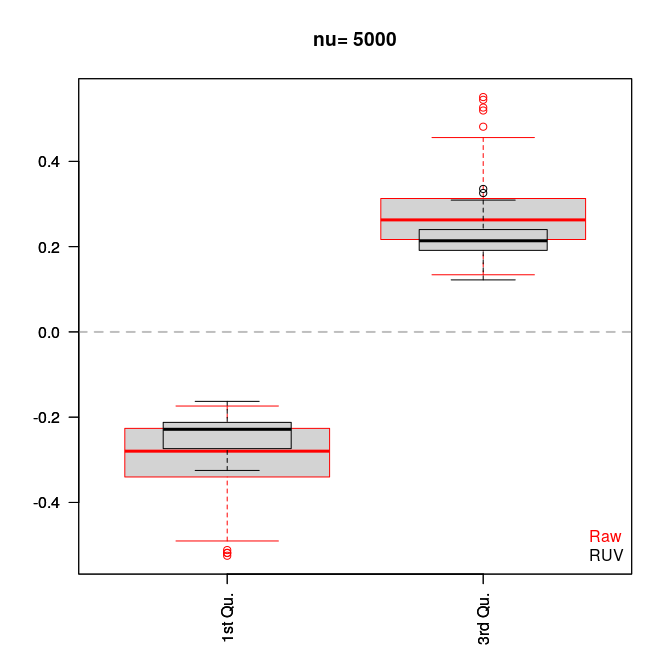## []
## NULL
##
## []
## NULL
##
## []
## NULL
##
## []
## NULL

The figure shows RLE plots comparing different options of $$\nu$$ for $$\hat{k}=3$$. The boxplots summarize the 25% and 75% quantile of all samples. The red boxplots display the raw data, while the black boxplots refer to the RUV applied with $$\hat{k}=2$$ and $$\nu$$ as in the title of the panel.

A parameter choice of $$\nu=500$$ seems to offer the best choice. In order to check whether the selected parameter at least removes all the known sources of variation, there is yet another version of the RLE plot. Here we plot the median and the inter-quantile-range (IQR) of the difference between the gene expression and the study median for all samples. Furthermore, it is useful to color these plots according to a known source of unwanted variation, such as batches.

The fugure shows RLE plots for data cleaned with RUV using $$\nu=500$$ for $$\hat{k}=2$$. Every sample is represented by the median and inter-quantile-range of the difference between observed gene expressions and study mean. The samples are colored according to their batches.

Principal component plots (PCAPlot) provide a similar way of assessing parameter choices for RUV.

The figure demonstrates that at least most of the systematic noise introduced via the batch effect has been removed. Hence, it is now possible to examine gene-gene correlations, construct gene networks or else using this new dataset.

CleanData <- expr_AllRUV[]

# Gene prioritisation

One of the methods that can be applied given a cleaned version of the dataset is gene prioritisation. Gene prioritisation identifies candidate genes that are likely to be involved in the same biological pathways or related pathways than a set of known genes. The gene prioritisation method in this package is very similar to the approach described in the paper by Oliver et al. For demonstration purposes assume that the following genes involved in the synaptic vesicle cycle are in fact candidates:

cand_genes <- c("CACNA1A", "CACNA1B", "SNAP25", "STX1A")
cand_affy <- names(which(unlist(lapply(xx, function(x) is.element(x, cand_genes)))))
cand_index <- which(is.element(colnames(CleanData),cand_affy))

## Finding the correlation threshold of significant co-expression

In order to prioritise genes, typically a correlation threshold is determined. The absolute values of correlations between genes that exceed this threshold are considered to be truly co-expressed. Here, we use a threshold that corresponds to a proportion of prioritised random genes of 0.3. However, this requires extensive estimation for all possible thresholds. This can be achieved using the function calculateThreshold:

Prop <- calculateThreshold(CleanData, exclude=c(nc_index, cand_index),
index.ref=na_index, set.size=length(cand_index),
Weights=NULL)
threshold <- predict(Prop$loess.estimate, 0.3) threshold ##  0.3722723 It is important to exclude genes that could bias the estimation of the proportion of prioritised genes. ## Prioritising candidate genes Having determined the threshold we can use the function prioritise in order to establish which candidates are also likely to be involved in the sodium-channel: prior<-prioritise(CleanData, na_index, cand_index, Weight=NULL, threshold=threshold) print(prior) ## prioritisedGenes strength strength2 ## 202507_s_at "202507_s_at" "2" "0.928856858297064" ## 206399_x_at "206399_x_at" "2" "0.943806430876702" xx[which(is.element(names(xx), prior[,1]))] ##$202507_s_at
##  "SNAP25"
##
## \$206399_x_at
##  "CACNA1A"

This analysis prioritises SNAP25 and CACNA1A.